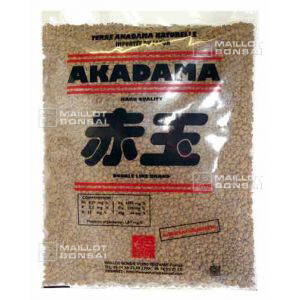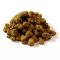##### The Japanese Maple specialist
Direct order Contact Help / Services Newsletter# Shelf to displaySearch

Japanese MaplesYoung trees Acer seeds and more Bonsai soil and fertiliserVarieties introduced into EuropeHostasWind bells and chimesSaxifragesSpecial offer

Packing and shipping charges

# Akadama bonsai drainage soil 2ltr bag big grain

› Bonsai soil and fertiliser › Bonsai soilref. : 5272

Product unavailable

###### Description

Large grain bonsai soil used for making drainage beds.

Small bag +- 2 litres. Weight +- 1.5 kilos bag. Grain size 3/5 mm. SUPERIOR QUALITY, DRIED FOR MORE THAN 2 YEARS. Weight according to the rate of moisture of 1.5 kilograms.

Origin: natural volcanic earth from the mountainous regions of Japan.

Benefits: soil drainage and root ventilation for the healthy growth of your bonsai.

How to use: use alone or add gravel and large-grained sand but not compost. This earth is not very nourishing and a Biogold fertiliser should be used regularly. You'll need to water this large-grained soil more in summer.

Species: for cultivating all coniferous and deciduous species (except rhododendrons and azaleas, for which you'll need Kanuma earth).

Please kindly note that this is a heavy product to send and we charge extra for postage.

#soil 7.4 #bonsai 6.6 #drainage 4.8 #grain 4.5 #large 3.5 #earth 3.5 #this 3.4 #grained 2.7 #species 2.7 #akadama 2.7

Formule
(( ROUND((CHAR_LENGTH(b.article_nom)-CHAR_LENGTH(REPLACE(b.article_nom, 'soil', '')))/LENGTH('soil')) + ROUND((CHAR_LENGTH(b.article_description)-CHAR_LENGTH(REPLACE(b.article_description, 'soil', '')))/LENGTH('soil')) ) * 5.4) + (( ROUND((CHAR_LENGTH(b.article_nom)-CHAR_LENGTH(REPLACE(b.article_nom, 'drainage', '')))/LENGTH('drainage')) + ROUND((CHAR_LENGTH(b.article_description)-CHAR_LENGTH(REPLACE(b.article_description, 'drainage', '')))/LENGTH('drainage')) ) * 4.8) + (( ROUND((CHAR_LENGTH(b.article_nom)-CHAR_LENGTH(REPLACE(b.article_nom, 'bonsai', '')))/LENGTH('bonsai')) + ROUND((CHAR_LENGTH(b.article_description)-CHAR_LENGTH(REPLACE(b.article_description, 'bonsai', '')))/LENGTH('bonsai')) ) * 4.6) + (( ROUND((CHAR_LENGTH(b.article_nom)-CHAR_LENGTH(REPLACE(b.article_nom, 'grain', '')))/LENGTH('grain')) + ROUND((CHAR_LENGTH(b.article_description)-CHAR_LENGTH(REPLACE(b.article_description, 'grain', '')))/LENGTH('grain')) ) * 4.5) + (( ROUND((CHAR_LENGTH(b.article_nom)-CHAR_LENGTH(REPLACE(b.article_nom, 'large', '')))/LENGTH('large')) + ROUND((CHAR_LENGTH(b.article_description)-CHAR_LENGTH(REPLACE(b.article_description, 'large', '')))/LENGTH('large')) ) * 3.5) + (( ROUND((CHAR_LENGTH(b.article_nom)-CHAR_LENGTH(REPLACE(b.article_nom, 'earth', '')))/LENGTH('earth')) + ROUND((CHAR_LENGTH(b.article_description)-CHAR_LENGTH(REPLACE(b.article_description, 'earth', '')))/LENGTH('earth')) ) * 3.5) + (( ROUND((CHAR_LENGTH(b.article_nom)-CHAR_LENGTH(REPLACE(b.article_nom, 'this', '')))/LENGTH('this')) + ROUND((CHAR_LENGTH(b.article_description)-CHAR_LENGTH(REPLACE(b.article_description, 'this', '')))/LENGTH('this')) ) * 3.4) + (( ROUND((CHAR_LENGTH(b.article_nom)-CHAR_LENGTH(REPLACE(b.article_nom, 'grained', '')))/LENGTH('grained')) + ROUND((CHAR_LENGTH(b.article_description)-CHAR_LENGTH(REPLACE(b.article_description, 'grained', '')))/LENGTH('grained')) ) * 2.7) + (( ROUND((CHAR_LENGTH(b.article_nom)-CHAR_LENGTH(REPLACE(b.article_nom, 'species', '')))/LENGTH('species')) + ROUND((CHAR_LENGTH(b.article_description)-CHAR_LENGTH(REPLACE(b.article_description, 'species', '')))/LENGTH('species')) ) * 2.7) + (( ROUND((CHAR_LENGTH(b.article_nom)-CHAR_LENGTH(REPLACE(b.article_nom, 'akadama', '')))/LENGTH('akadama')) + ROUND((CHAR_LENGTH(b.article_description)-CHAR_LENGTH(REPLACE(b.article_description, 'akadama', '')))/LENGTH('akadama')) ) * 2.7)

## Secure payment## Delivery

Our logistic partners :04 74 55 23 48
Pépinière MAILLOT-BONSAÏ
Le Bois Frazy
01990 RELEVANT - FRANCE
on appointment